﻿ Maximum Power Point Tracking for Solar Photovoltaic System Using Genetic Programming Toolbox for Identification of Physical System

### Maximum Power Point Tracking for Solar Photovoltaic System Using Genetic Programming Toolbox for Ide...OPEN ACCESSPEER-REVIEWED

## Maximum Power Point Tracking for Solar Photovoltaic System Using Genetic Programming Toolbox for Identification of Physical System

Sajjad Aliesfahani1,, Mehdi Shahbazian1

1Department of Instrumentation and Automation Engineering, Petroleum University of Technology, Ahwaz, Iran

### Abstract

Due to the increasing demands for energy in recent years and declining fossil fuel resources and the pollution that these energy sources are created, the solar energy produced by sun, has attracted much attention. Solar cells are elements that convert the solar energy into electricity. One of the challenges that we encounter is to obtain the most of the solar resource potential in various conditions of radiation, temperature and wind speed. A major task is to find an efficient and fast algorithm for tracking the maximum power point. In this paper, a new method for maximum power point tracking of photovoltaic (PV) cell that based on genetic programming toolbox of identification of physical system (GPTIPS), has been proposed. The maximum values of the voltage and the current of the solar cell is predicted by the GPTIPS algorithm and then the optimum duty cycle is produced for the chopper. Which cause maximum power deliver to the load. It was observed that the mean square error is reached to order of 10-5 approximately, that in similar circumstances, the mean square error and offline training time is less than a multi-layer perceptron of neural network. Simulation results show that the proposed GPTIPS approach is superior to the neural network method.

### At a glance: Figures

123
Prev Next

• Aliesfahani, Sajjad, and Mehdi Shahbazian. "Maximum Power Point Tracking for Solar Photovoltaic System Using Genetic Programming Toolbox for Identification of Physical System." Journal of Automation and Control 3.1 (2015): 25-28.
• Aliesfahani, S. , & Shahbazian, M. (2015). Maximum Power Point Tracking for Solar Photovoltaic System Using Genetic Programming Toolbox for Identification of Physical System. Journal of Automation and Control, 3(1), 25-28.
• Aliesfahani, Sajjad, and Mehdi Shahbazian. "Maximum Power Point Tracking for Solar Photovoltaic System Using Genetic Programming Toolbox for Identification of Physical System." Journal of Automation and Control 3, no. 1 (2015): 25-28.

 Import into BibTeX Import into EndNote Import into RefMan Import into RefWorks

### 1. Introduction

Due to depleting fossil fuel resources and the pollution that these are transfer into the environment, tend to produce clean and renewable energy is much higher. one of these energy sources is solar energy that is always available in everywhere. [1, 2] Solar cells are components that produce electricity by solar energy. Performance of this elements is affected by environmental factors such as radiation, air temperature and wind speed. Voltage, current and power of solar cells varies nonlinearity with changing environmental conditions. [3, 4] It is desirable that at any moment and in any circumstances, the maximum power generated by the solar cell and transfer to load. several of algorithms available for the MPPT including perturb and observe, incremental conductance, parasitic capacitance, constant voltage and fuzzy logic algorithms [5, 6, 7, 8]. These methods have disadvantages like costly, difficult to implement and non-stable. For this purpose genetic programming toolbox for identification of physical system (GPTIPS) come with a solution. GPTIPS sometimes (usually when only a few input variables are involved) lags behind a neural network model in terms of raw predictive performance but the equivalent GP models are often simpler, shorter and may be open to physical interpretation. It's not always an easy question to answer.

At any rate, on practical applications GPTIPS normally significantly outperforms neural network models in terms of model performance, interpretability, deployability and robustness.also GPTIPS is better than the PLS. In my opinion, PLS regression is an overused and overvalued analytical tool. PLS regression is also hard to understand (well at least I find it hard to understand) and the models it produces are complex, fragile, hard to deploy and difficult to interpret.For impedance matching and obtain maximum power, a dc-dc converter located between the solar cell and load. A maximum power point tracker required for optimal control of duty cycle of dc-dc converter. [9, 10] There are many techniques for maximum power point tracking. In this paper for generation and control the duty cycle used GPTIPS. It has several advantages such as high accuracy and faster performance compared to neural network and fuzzy logic control. [11, 12] View block diagram of the solar photovoltaic system, we can see the Figure 1.

### 2. Model of Solar PV Cell

A simple model of a PV cell is shown as an equivalent circuit in Figure 2 that consists of an ideal current source in parallel with an ideal diode, with a parallel shunt resistance Rsh and series resistance Rs [13, 14].

Based on the equivalent circuit, the current to the load can be given as [15, 16, 17]: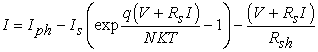(1)

In this equation,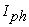, is the photocurrent,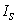, is the reverse saturation current of the diode, q is the electron charge, V is the voltage across the diode, K is the Boltzmann's constant, T is the junction temperature, N is the ideality factor of the diode, and Rs and Rsh are the series and shunt resistors of the cell, respectively. As a result, the complete physical behavior of the PV cell depends on,, Rs and Rsh from one hand and with three environmental parameters as the temperature, the solar radiation and wind speed on the other hand. The above equation is a function of two equations:

One that calculates the PV cell photocurrent which depends on the radiation and the temperature according to equation .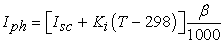(2)

Where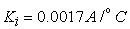is the cell's short circuit current temperature coefficient,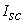short circuit current of solar cell (A) and β is the solar radiation (W/m). The actual cell temperature (T) has been found to be dependent on ambient air temperature, radiation and wind speed, which has been modeled by the following relationship :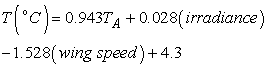(3)

Where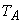is the ambient air temperature given in °C, irradiance in W/m2 and wind speed in m/s. The above solar PV cell model has been implemented in MATLAB and the model’s accuracy is also validated by a comparison between it and datasheet of Q6LMXP3-G3.

Simulated P-V and I-V characteristics of solar cell are shown in Figure 3.

Figure 3. Comparison of the simulated P-V and I-V characteristics of solar cell at irradiance level 1000 W/m2 and ambient air temperature 25°C and the Data sheet of Q6LMXP3-G3

### 3. Methodology and MPPT Controller

Genetic programming is an approach to identification and estimation the output of a system with respect to itself inputs. In this method, set the number of generations and functions that are used to identify the system will be done. Then having the inputs and output data, mathematical model of the system is constructed by this method. In fact, a function or a equation is produced respect to inputs and output of the system. According to this equation, the output of the system with respect to new inputs can be estimated. It means after training the system, the output can be achieved in a short time. .

#### Table 1. comparison between GPTIPS & NNDownload asPowerPoint Slide

Veiw figureView current table in a new window

The model uses the cell operating temperature and radiation as input values and estimates the corresponding maximum power voltage and current values. In Figure the actual data values and the GPTIPS-estimated values of maximum power current Imax and voltage Vmax for training data and testing data have been compared. The values Imax and Vmax correspond to solar intensity variation between 100 and 1000 W/m2 and the cell temperatures of 10 to 50°C, whose ranges are relevant to practical situation. One can notice the good agreement between all sets of results. It was observed that the mean square error is reached to order of 10-5 approximately, that in similar circumstances, the mean square error and offline training time is less than a multi-layer perceptron of neural network. See Table 1 and Figure 4 and Figure 5.

Figure 4. Comparison of actual max power current and voltage values with the maximum power current and voltage values obtained by GPTIPS predictor.

### 4. Extract of Maximum Power

A buck & boost chopper is used for impedance matching between the solar cell and load. The GPTIPS predictor estimate maximum power current and maximum power voltage and then the optimum duty cycle generates by using equation (1) for feeding the chopper.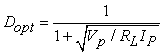(4)

### 5. Results and Discussion

Output voltage, current and power at constant atmospheric temperature and wind speed with different irradiation display of the SIMULINK model of the complete SPV system is illustrated in Figure 6.

### 6. Simulation of Maximum Power Point Tracker Using SIMULINK

A SIMULINK model of a complete SPV system is shown in Figure 7 realizing the LSSVM based maximum power point controller is. This model consists of six subsystems, namely Inputs block, SPV cell Model, prediction system, Dopt block, PWM block and Chopper. The Inputs subsystem contains the input parameters for the SPV cell subsystem are incident solar radiation; atmospheric temperature and wind speed. The SPV cell Model subsystem is characterized by Eqs. (1)-(3); The predictor system subsystem gives the maximum voltage and current for all practical values of radiation and temperature. The Dopt block calculates the optimum duty cycle using Eq. (4) and it produces into the PWM subsystem. The variation of maximum power output Pmax corresponding atmospheric ambient conditions is shown in Figure 8.

A ramp load has been considered to show that the optimum power delivered is constant at particular radiation and temperature at variable conditions of load.

Figure 8. The variation of maximum power output Pmax corresponding the variation of irradiation and constant temperature and wind speed

### 7. Conclusion

A new method based on the genetic programming for MPPT of PV energy harvesting system has been developed. A complete PV system with GPTIPS MPPT controller was modeled in MATLAB/Simulink Simulation It was observed that the mean square error is reached to order of 10-5 approximately, that in similar circumstances, the mean square error and offline training time is less than a multi-layer perceptron of neural network. results show that the MPPT controller using the GPTIPS method is robust and accurate compared to the other MPPT methods and that it successfully tracks the global maximum power point of a PV module. This system can be applied in the building integrated PV or grid connected PV systems.

### Nomenclature

A: Ampere

D: duty cycle of the converter

Dopt: optimum duty cycle

E: electron charge 1.6X10-19C

Eg: energy band gap (eV)

ID: diode current (A)

Iin: converter input current (A)

Imax: maximum power current (A)

IP: current estimated by LSSVM predictor corresponding to peak power point (A)

Is(t): reverse saturation current (A)

Is: reverse saturation current reference (A)

Iout: converter output current (A)

Iph: photo-generated current (A)

Ish: shunt current (A)

K: Boltzmann constant, 1.38X10-23J/K

RLin: input resistance of the converter (Ω)

Rs: series resistance (Ω)

Rsh: shunt resistance (Ω)

TA: ambient air temperature (1C)

V: voltage

Vmax: maximum power voltage (volt)

Vin: converter input voltage (volt)

VP: voltage estimated by LSSVM predictor corresponding to peak power point (volt)

Vout: converter output voltage (volt)

N: diode ideality factor

### References

  F. Blaabjerg, Z. Chen, and R. Teodorescu, “Renewable energy systems in the power electronics curriculum,” in Power Electronics Education, 2005. IEEE Workshop, 2005, pp. 58-68.In article  T. Khatib, A. Mohamed, and K. Sopian, “Optimization of a PV/wind micro-grid for rural housing electrification using a hybrid iterative/genetic algorithm: case study of Kuala Terengganu, Malaysia,” Energy and Buildings, vol. 47, pp. 321-331, 2012.In article  D. Furkan and M. Mehmet Emin, “Critical factors that affecting efficiency of solar cells,” Smart Grid and Renewable Energy, vol. 2010, 2010.In article  T. Esram and P. L. Chapman, “Comparison of photovoltaic array maximum power point tracking techniques,” IEEE TRANSACTIONS ON ENERGY CONVERSION EC, vol. 22, p. 439, 2007.In article  C. Hua and C. Shen, “Comparative study of peak power tracking techniques for solar storage system,” in Applied Power Electronics Conference and Exposition, 1998. APEC'98. Conference Proceedings 1998., Thirteenth Annual, 1998, pp. 679-685.In article  K. Hussein, I. Muta, T. Hoshino, and M. Osakada, “Maximum photovoltaic power tracking: an algorithm for rapidly changing atmospheric conditions,” IEE Proceedings-Generation, Transmission and Distribution, vol. 142, pp. 59-64, 1995.In article  R. Ramaprabha and B. Mathur, “Intelligent controller based maximum power point tracking for solar PV system,” International Journal of Computer Applications, vol. 12, pp. 37-42, 2011.In article  M. M. Algazar, H. Al-Monier, H. A. EL-halim, and M. E. E. K. Salem, “Maximum power point tracking using fuzzy logic control,” International Journal of Electrical Power & Energy Systems, vol. 39, pp. 21-28, 2012.In article  C.-F. Lu, C.-C. Liu, and C.-J. Wu, “Dynamic modelling of battery energy storage system and application to power system stability,” IEE Proceedings-Generation, Transmission and Distribution, vol. 142, pp. 429-435, 1995.In article  A. F. Boehringer, “Self-Adapting dc Converter for Solar Spacecraft Power Supply Selbstanpassender Gleichstromwandler fÃ¼r die Energieversorgung eines Sonnensatelliten,” Aerospace and Electronic Systems, IEEE Transactions on, pp. 102-111, 1968.In article  H. J. Möller, Semiconductors for solar cells: Artech House Boston^ eMA MA, 1993.In article  R. Gottschalg, M. Rommel, D. Infield, and H. Ryssel, “Comparison of different methods for the parameter determination of the solar cell's double exponential equation,” in Proceedings of the 14th European Photovoltaic Solar Energy Conference (PVSEC), Barcelona, Spain, 1997, pp. 321-324.In article  F. M. González-Longatt, “Model of photovoltaic module in Matlab,” II CIBELEC, vol. 2005, pp. 1-5, 2005.In article  H.-L. Tsai, C.-S. Tu, and Y.-J. Su, “Development of generalized photovoltaic model using MATLAB/SIMULINK,” in Proceedings of the world congress on Engineering and computer science, 2008, pp. 1-6.In article  I. Altas and A. Sharaf, “A photovoltaic array simulation model for Matlab-Simulink GUI environment,” in Clean Electrical Power, 2007. ICCEP'07. International Conference on, 2007, pp. 341-345.In article  K. Kachhiya, M. Lokhande, and M. Patel, “MATLAB/Simulink model of solar PV module and MPPT algorithm,” in Proceedings of the National Conference on Recent Trends in Engineering and Technology, 2011.In article  T. Salmi, M. Bouzguenda, A. Gastli, and A. Masmoudi, “Matlab/simulink based modeling of photovoltaic cell,” International Journal of Renewable Energy Research (IJRER), vol. 2, pp. 213-218, 2012.In article  S. Nema, R. Nema, and G. Agnihotri, “MATLAB/Simulink based study of photovoltaic cells/modules/array and their experimental verification,” International journal of Energy and Environment, vol. 1, pp. 487-500, 2010.In article  R. Chenni, M. Makhlouf, T. Kerbache, and A. Bouzid, “A detailed modeling method for photovoltaic cells,” Energy, vol. 32, pp. 1724-1730, 2007.In article  D. P. Searson, D. E. Leahy, and M. J. Willis, “GPTIPS: an open source genetic programming toolbox for multigene symbolic regression,” in Proceedings of the International multiconference of engineers and computer scientists, 2010.In article  N. Mohan and T. M. Undeland, Power electronics: converters, applications, and design: John Wiley & Sons, 2007.In article